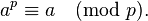Hackerrank Nov Challenge – Power of Large Numbers

PROBLEM STATEMENT

In a nutshell, we have a^b mod 10^9 + 7 ( ^ denotes power )

Range of a & b: 1 <= a,b <= 10^100000

PRE – REQUISITES

FERMAT’S THEOREM:

The theorem stats that, if is a prime number, then for any arbitrary integer